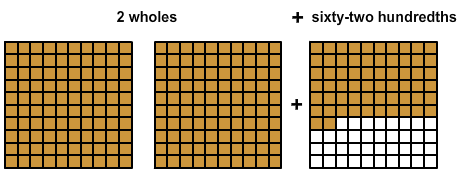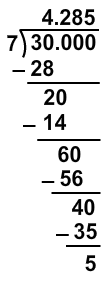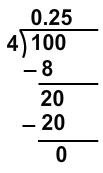# Converting Mixed Numbers to Decimals

Rate Lesson 0 stars
Lesson size:
Message preview:
Someone you know has shared lesson with you:

To play this lesson, click on the link below:

https://www.turtlediary.com/lesson/converting-mixed-numbers-to-decimals.html

Hope you have a good experience with this site and recommend to your friends too.

Login to rate activities and track progress.
Login to rate activities and track progress.

If you can convert a fraction to a decimal, you can easily convert a mixed number, also called a mixed fraction, to a decimal.

A mixed number is a whole number as well as some parts of a whole as fraction.

For example, 2
62
100
or two and sixty-two hundredths means there are two whole parts and an additional sixty-two out of one hundred parts.When you convert a mixed fraction to a decimal, there are two strategies:

• Change the mixed fraction to an improper fraction and then divide.
• Convert the fractional part only then add it to the whole number. In other words, just leave the whole number the same and convert the fraction.

Let's take a look at some examples.

## Example 1

Write 4
2
7
as a decimal.

First change the mixed fraction to an improper fraction.

4
2
7
= 4 +
2
7
=
4
1
+
2
7
=
4 x 7
1 x 7
+
2
7
=
28
7
+
2
7
=
28 + 2
7
=
30
7

Now divide 7 into 30.

Since 30 is greater than 7, the quotient will be a whole number and a decimal.Round the decimal to the nearest hundredth.

So, 4
2
7
= 4.29

## Example 2

Write 12
3
5
as a decimal.

First change the mixed fraction to an improper fraction.

12
3
5
= 12 +
3
5
=
12
1
+
3
5
=
12 x 5
1 x 5
+
3
5
=
60
5
+
3
5
=
60 + 3
5
=
63
5

Now divide 5 into 63.So, 12
3
5
= 12.6

## Example 3

Write 3
4
10
as a decimal.
First convert the fractional part
4
10
to decimal 0.4.

Now add 0.4 to 3 .

3
4
10
= 3 + 0.4 = 3.4
So, 3
4
10
= 3.4

## Example 4

Write 6
1
4
as a decimal.

First convert the fractional part by dividing 4 into 1.1 ÷ 4 = 0.25

6
1
4
= 6 +
1
4
= 6 + 0.25 = 6.25
So, 6
1
4
= 6.25
Summary
• A mixed number is a whole number as well as some parts of a whole as fraction.
• We can convert a mixed number (or mixed fraction) to a decimal.
• Strategies to convert mixed fractions to decimals:
• Change the mixed fraction to an improper fraction and then divide.
• Convert the fractional part only and then add it to the whole number.

## More Decimals Games

Become premium member to get unlimited access.